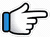# Ek Haseena Thi Ek Deewana Tha – Keyboard Notes## Ek Haseena Thi Ek Deewana Tha Keyboard NotesFor Ek Haseena Thi Ek Deewana Tha Notes as Western or Indian Notations, Click –

Western –
Indian –

Lyrics –

## Keyboard Notes for Ek Haseena Thi Ek Deewana Tha – Indian Notation (srg mpdn)

s*      n  d  n       D
Ek     haseena      thi

n       d  D d        m
ek     deewana     tha

g        m r      m      p  g
Kya   umar   kya    samaa

d      D d  n     s*      r* s*
kya  zamana  tha     ~ ~

s*       n  d  n      D
Ek      hasina     thi

n  d  n       D
hasina      thi

n       d  D d         m
Ek     deewana    tha

d    D d        m
deewana     tha

g      m r       m      p  g
Kya  umar    kya   samaa

d      D d  n      s*      r* s*
kya  zamana    tha   ~~

g g    m p    p       m  m
Ek    din     woh  mile

r  r      r m m      g  g
roz     milne       lage

g g   m p    p       m m   d m
Ek    din    who   mile    ~~

r  r     r  m m      g  g
roz    milne       lage

g        g  p ~ p        m  m
Phir   mohabbat     huyi

r         r  r m m      g  g
bas   qayamat      huyi

d       D d      n       n  s*
Kho  gaye    tum   kahan

d  D        d      n  n s*
sunke    yeh   dastaan

d  D     d    n  n     s*
Log     hairaan     hain

d  D       d  n        n  s*
kyunki    anjaan    hain

s* n     d     p     m  m  mds
Ishq    ki    who  gali     ~~

s*  d       m       p m    g
baat      jiski     chali

n       d  n     s*
Us    gali     mein

n  d       n  s*    n d        d
mera    aana    jaana    tha
Or
n  d       n  s*    n d n    s*  d

d    D d  n    D
Ek  hasina    thi

D d     n   n  d     d
ek      deewana  tha

n       d n        s*
Kya  umar    thi

n      d  n      s*
kya  samaa  tha

n      d  n  s*    d
kya  zamana  tha

d      D d  n     D
Ek  hasina     thi

D d     n  n ~  d      d
ek      deewana    tha

g        g m g     r      s  r
Us     hasin     ne    kaha

g  g       m g  r      s  r
suno     jaane     wafa

g         g  m g     r      s  r
Yeh    falak     yeh    zameen

g  g     m g   r            s  r
tere    bin     kuch    nahin

d D         d g m    g        g
Tujhpe   marti     hoon  main

d D      d g m    g      g
pyaar   karti     hoon  main

d D       d g     m g      g
Baat      kuch    aur      thi

d        D d g      m g      g
woh    nazar     chor    thi

s*  n    d   p       m m~   m d s*
Uske   dil  mein  chupi   ~~

s* d      m p     m    g
chaah  daulat   ki   thi

n d      n     s*    n  s*~
Pyaar  ka   woh  fakat
n d      n     s*    n d  n  s*

n    d n  s*    d
ek  bahana  tha

d    D d  n     D
Ek  hasina      thi

D d     n    n  d    d
ek      deewana  tha

n        d n       s*
Kya    umar   thi

n      d  n         s*
kya  samaa    tha

n         d  n  s*    d
kya    zamana   tha

d      D d  n     D
Ek    hasina     thi

D d    n    n  d    d
ek     deewana  tha

## Song Information of Ek Haseena Thi Ek Deewana Tha

Song: Ek Haseena Thi Ek Deewana Tha
Movie: Karz (1980)
Singer: Kishore Kumar, Asha Bhosle
Music: Laxmikant Pyarelal
Lyrics: Anand Bakshi